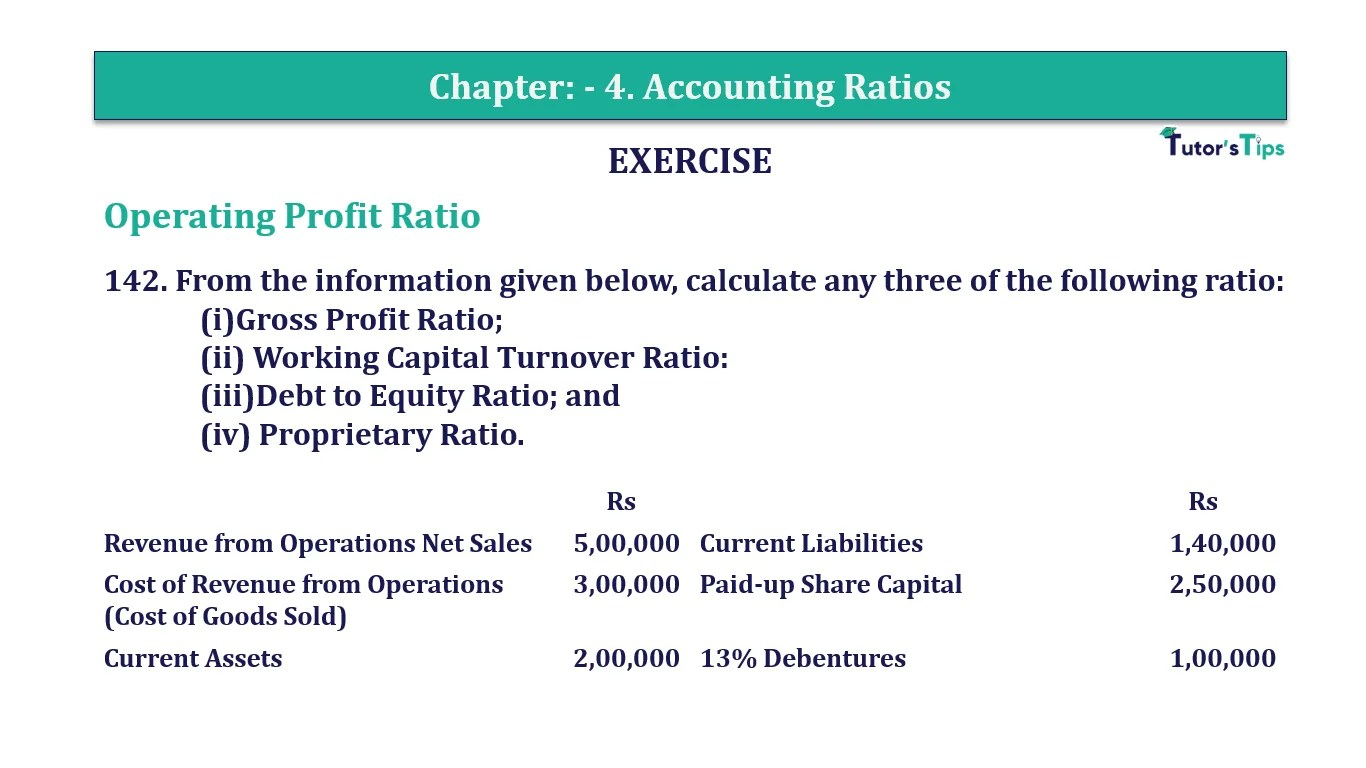# Question 142 Chapter 4 of +2-B – T.S. Grewal 12 ClassQuestion No. 142- Chapter No.4 - T.S. Grewal +2 Book Part B

Question 142 Chapter 4 of +2-B

Miscellaneous

142. From the information given below, calculate any three of the following ratio:
(i)Gross Profit Ratio;
(ii) Working Capital Turnover Ratio:
(iii)Debt to Equity Ratio; and
(iv) Proprietary Ratio.

 Rs Rs Revenue from Operations Net Sales 5,00,000 Current Liabilities 1,40,000 Cost of Revenue from Operations (Cost of Goods Sold) 3,00,000 Paid-up Share Capital 2,50,000 Current Assets 2,00,000 13% Debentures 1,00,000

### The solution of Question 142 Chapter 4 of +2-B: –

I

 Net Sales = Rs 5,00,000 Cost of Goods Sold = Rs 35,000 Gross Profit = Net Sales − Cost of Goods Sold = Rs 5,00,000 – Rs 3,00,000 = Rs 2,00,000
 Gross Profit Ratio = Gross Profit X 100 Net Sales
 Gross Profit Ratio = Rs 2,00,000 X 100 Rs 5,00,000 = 40%

II

 Net Sales = Rs 1,20,000 Operating Cost = Cost of Goods Sold + Operating Expenses = Rs 60,000 + Rs 40,000 = Rs 1,00,000
 Operating Ratio = Operating Cost X 100 Net Sales
 Operating Ratio = Rs. 1,00,000 X 100 Rs 1,20,000 = 83.33%

III

 Long-term Debts = Rs 1,00,000 Equity = Rs 2,50,000
 Debt – Equity Ratio = Long-term Debts Equity
 Debt – Equity Ratio = Rs. 1,00,000 Rs 2,50,000 = 0.4 : 1

IV

 Total Assets = Total Liabilities Operating Cost = Current Liabilities + Paid-up Share Capital + 13% Debentures = Rs 1,40,000 + Rs 2,50,000 + Rs 1,00,000 = Rs 4,90,000
 Proprietary Ratio = Shareholders’ Fund Total Assets
 Proprietary Ratio = Rs. 2,50,000 Rs 4,90,000 = 0.51 : 1

Comment if you have any question.

Also, Check out the solved question of previous Chapters: –